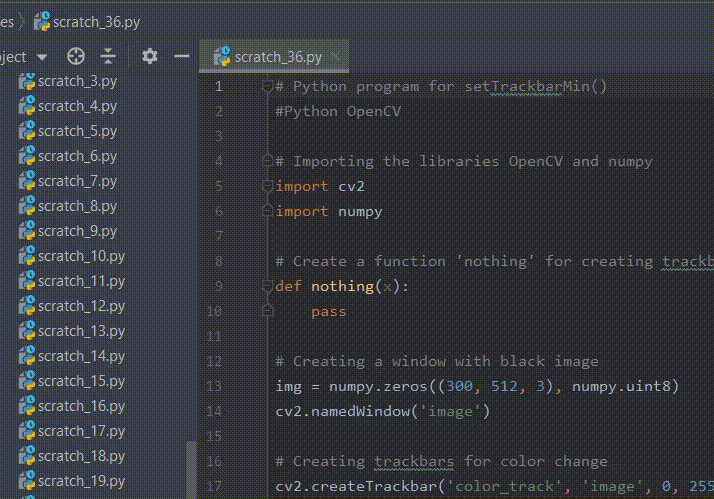Open in App
Not now

# Python OpenCV – setTrackbarMin()

• Last Updated : 03 Jan, 2023

In this article, we will discuss how to set the minimum position of the trackbar. The minimum value of the track bar can be achieved using the function setTrackbarMin() from the OpenCV package in python. This function sets the minimum position of the specified trackbar in the specified window after creating the trackbar.

## setTrackbarMin() function:

setTrackbarMin() function sets the minimum position of the track bar in the window. It does not return anything. This function takes three arguments. The first is for the trackbar name and the second one is the window name which is the parent of the trackbar and the third one is for the new value of the position that is to be set to the trackbar. It returns None.

Syntax: cv.setTrackbarMax(trackbarname, winname, maxval)

Parameters:

• trackbarname: Name of the trackbar
• winname: Name of the window that is the parent of trackbar
• maxval: New maximum position

Return: None

Example 1:

In this example, we have created a window with a black image. Further, we create a trackbar using the createTrackbar() function and set the minimum value of trackbar using setTrackbarMin() function to 100. After that, we will create a loop for displaying an image and trackbar. So, whenever we move the trackbar position, the shades of black change.

## Python3

 `# Python program for setTrackbarMin() ` `# Python OpenCV ` ` `  `# Importing the libraries OpenCV and numpy ` `import` `cv2 ` `import` `numpy ` ` `  `# Create a function 'nothing' for ` `# creating trackbar ` `def` `nothing(x): ` `    ``pass` ` `  `# Creating a window with black image ` `img ``=` `numpy.zeros((``300``, ``512``, ``3``), numpy.uint8) ` `cv2.namedWindow(``'image'``) ` ` `  `# Creating trackbars for color change ` `cv2.createTrackbar(``'color_track'``, ``'image'``, ``0``, ``255``, nothing) ` ` `  `# Setting minimum position of 'color_track'  ` `# trackbar to 100 ` `cv2.setTrackbarMin(``'color_track'``, ``'image'``, ``100``) ` ` `  `# Create a loop for displaying image and  ` `# trackbar ` `while``(``True``): ` ` `  `    ``# Display the image ` `    ``cv2.imshow(``'image'``, img) ` ` `  `    ``# Create a button for pressing and changing ` `    ``# the window ` `    ``k ``=` `cv2.waitKey(``1``) & ``0xFF` `    ``if` `k ``=``=` `27``: ` `        ``break` ` `  `    ``# Get current positions of trackbar ` `    ``color ``=` `cv2.getTrackbarPos(``'color_track'``, ``'image'``) ` ` `  `    ``# Display color mixture ` `    ``img[:] ``=` `[color] ` ` `  `# Close the window ` `cv2.destroyAllWindows()`

Output:Example 2:

In this example, we have created a window with a black image. Further, we create three trackbars of red, green, and blue color using the createTrackbar() function and set the minimum values of the trackbar using the setTrackbarMin() function to 50, 100, and 150. Later, we create a loop for displaying an image and trackbar. So, whenever we move the trackbar positions, the shades of red, green, and blue change.

## Python3

 `# Python program for setTrackbarMin() ` `#Python OpenCV ` ` `  `# Importing the libraries OpenCV and numpy ` `import` `cv2 ` `import` `numpy ` ` `  `# Create a function 'nothing' for  ` `# creating trackbar ` `def` `nothing(x): ` `    ``pass` ` `  `# Creating a window with black image ` `img ``=` `numpy.zeros((``300``, ``512``, ``3``), numpy.uint8) ` `cv2.namedWindow(``'image'``) ` ` `  `# Creating trackbars for red color change ` `cv2.createTrackbar(``'Red'``, ``'image'``, ``0``, ``255``, nothing) ` ` `  `# Creating trackbars for Green color change ` `cv2.createTrackbar(``'Green'``, ``'image'``, ``0``, ``255``, nothing) ` ` `  `# Creating trackbars for Blue color change ` `cv2.createTrackbar(``'Blue'``, ``'image'``, ``0``, ``255``, nothing) ` ` `  `# Setting minimum values of green color trackbar ` `cv2.setTrackbarMin(``'Green'``, ``'image'``, ``50``) ` ` `  `# Setting minimum values of red color trackbar ` `cv2.setTrackbarMin(``'Red'``, ``'image'``, ``100``) ` ` `  `# Setting minimum values of blue color trackbar ` `cv2.setTrackbarMin(``'Blue'``, ``'image'``, ``150``) ` ` `  `# Create a loop for displaying image and trackbar ` `while``(``True``): ` ` `  `    ``# Display the image ` `    ``cv2.imshow(``'image'``, img) ` ` `  `    ``# Create a button for pressing and  ` `    ``# changing the window ` `    ``k ``=` `cv2.waitKey(``1``) & ``0xFF` `    ``if` `k ``=``=` `27``: ` `        ``break` ` `  `    ``# Get current positions of red color trackbar ` `    ``red_color ``=` `cv2.getTrackbarPos(``'Red'``, ``'image'``) ` ` `  `    ``# Get current positions of green color trackbar ` `    ``green_color ``=` `cv2.getTrackbarPos(``'Green'``, ``'image'``) ` ` `  `    ``# Get current positions of blue color trackbar ` `    ``blue_color ``=` `cv2.getTrackbarPos(``'Blue'``, ``'image'``) ` ` `  `    ``# Display color mixture ` `    ``img[:] ``=` `[blue_color, green_color, red_color] ` ` `  `# Close the window ` `cv2.destroyAllWindows()`

Output:My Personal Notes arrow_drop_up
Related Articles